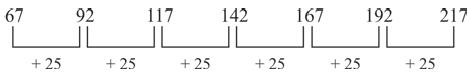Courses

# Olympiad Test: Series Completion - 2

## 10 Questions MCQ Test Science Olympiad Class 8 | Olympiad Test: Series Completion - 2

Description
This mock test of Olympiad Test: Series Completion - 2 for Class 8 helps you for every Class 8 entrance exam. This contains 10 Multiple Choice Questions for Class 8 Olympiad Test: Series Completion - 2 (mcq) to study with solutions a complete question bank. The solved questions answers in this Olympiad Test: Series Completion - 2 quiz give you a good mix of easy questions and tough questions. Class 8 students definitely take this Olympiad Test: Series Completion - 2 exercise for a better result in the exam. You can find other Olympiad Test: Series Completion - 2 extra questions, long questions & short questions for Class 8 on EduRev as well by searching above.
QUESTION: 1

### Find the next term in the following terms. 5, 24, 61, 122, 213, ?

Solution:

5   24   61   122   213   340

QUESTION: 2

### Find the next term in the following terms. 2, 5, 9, 14, 20, ?

Solution: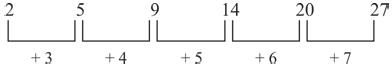QUESTION: 3

### Find the next term in the following terms. 28, 33, 31, 36, 34, ?

Solution: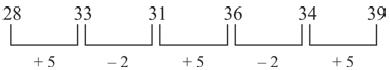QUESTION: 4

Find the next term in the following terms.
3, 4, 10, 33, 136, ?

Solution: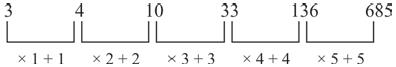QUESTION: 5

Find the next term in the following terms.
25, 49, 81, 121, 169, 225, ?

Solution: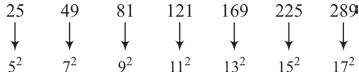QUESTION: 6

Find the next term in the following terms.
9, 27, 31, 155, 161, ?

Solution: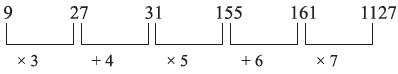QUESTION: 7

Find the next term in the following terms.
12, 23, 45, 78, 112, ?

Solution: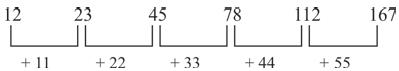QUESTION: 8

Find the next term in the following terms.
2, 3, 8, 27, 112, ?

Solution: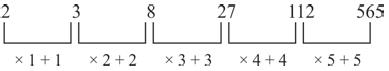QUESTION: 9

Find the next term in the following terms.
45, 68, 91, 114, ?

Solution: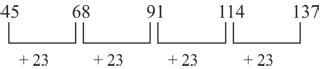QUESTION: 10

Find the next term in the following terms.
67, 92, 117, 142, 167, 192, ?

Solution: